Home
Hostname: page-component-99c86f546-45s75 Total loading time: 0.181 Render date: 2021-12-05T06:40:17.603Z Has data issue: true Feature Flags: { "shouldUseShareProductTool": true, "shouldUseHypothesis": true, "isUnsiloEnabled": true, "metricsAbstractViews": false, "figures": true, "newCiteModal": false, "newCitedByModal": true, "newEcommerce": true, "newUsageEvents": true }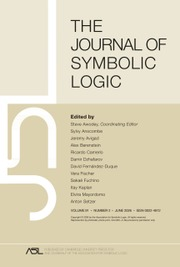The Journal of Symbolic Logic

# THE EXACT STRENGTH OF THE CLASS FORCING THEOREM

Part of: Set theory

Published online by Cambridge University Press:  29 July 2020

## Abstract

The class forcing theorem, which asserts that every class forcing notion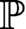${\mathbb {P}}$ admits a forcing relation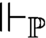$\Vdash _{\mathbb {P}}$ , that is, a relation satisfying the forcing relation recursion—it follows that statements true in the corresponding forcing extensions are forced and forced statements are true—is equivalent over Gödel–Bernays set theory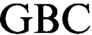$\text {GBC}$ to the principle of elementary transfinite recursion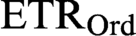$\text {ETR}_{\text {Ord}}$ for class recursions of length$\text {Ord}$ . It is also equivalent to the existence of truth predicates for the infinitary languages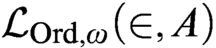$\mathcal {L}_{\text {Ord},\omega }(\in ,A)$ , allowing any class parameter A; to the existence of truth predicates for the language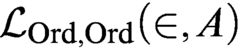$\mathcal {L}_{\text {Ord},\text {Ord}}(\in ,A)$ ; to the existence of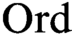$\text {Ord}$ -iterated truth predicates for first-order set theory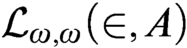$\mathcal {L}_{\omega ,\omega }(\in ,A)$ ; to the assertion that every separative class partial order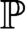${\mathbb {P}}$ has a set-complete class Boolean completion; to a class-join separation principle; and to the principle of determinacy for clopen class games of rank at most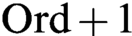$\text {Ord}+1$ . Unlike set forcing, if every class forcing notion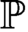${\mathbb {P}}$ has a forcing relation merely for atomic formulas, then every such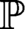${\mathbb {P}}$ has a uniform forcing relation applicable simultaneously to all formulas. Our results situate the class forcing theorem in the rich hierarchy of theories between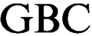$\text {GBC}$ and Kelley–Morse set theory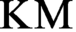$\text {KM}$ .

## MSC classification

Type
Articles
Information
The Journal of Symbolic Logic , September 2020 , pp. 869 - 905
© The Association for Symbolic Logic 2020

## Access options

Get access to the full version of this content by using one of the access options below. (Log in options will check for institutional or personal access. Content may require purchase if you do not have access.)

## References

Fujimoto, K., Classes and truths in set theory . Annals of Pure and Applied Logic , vol. 163 (2012), no. 11, pp. 14841523.CrossRefGoogle Scholar
Gitman, V. and Hamkins, J. D., Open determinacy for class games , Foundations of Mathematics (Caicedo, A. E., Cummings, J., Koellner, P., and Larson, P., editors), Contemporary Mathematics, vol. 690, American Mathematical Society, Providence, RI (2017) Newton Institute, 2016, pp. 121143.CrossRefGoogle Scholar
Hamkins, J. D. and Leahy, C., Algebraicity and implicit definability in set theory . Notre Dame Journal of Formal Logic , vol. 57 (2016), no. 3, pp. 431439.CrossRefGoogle Scholar
Hamkins, J. D. and Seabold, D., Well-founded Boolean ultrapowers as large cardinal embeddings, preprint, 2006, arXiv:1206.6075.Google Scholar
Hamkins, J. D. and Yang, R., Satisfaction is not absolute. The Review of Symbolic Logic , to appear. Preprint, arXiv:1312.0670 (2013).Google Scholar
Holy, P., Krapf, R., Lücke, P., Njegomir, A., and Schlicht, P., Class forcing, the forcing theorem and Boolean completions, this Journal, vol. 81 (2016), no. 4, pp. 15001530.Google Scholar
Holy, P., Krapf, R., and Schlicht, P., Characterizations of pretameness and the Ord-cc . Annals of Pure and Applied Logic , vol. 169 (2018), no. 8, pp. 775802.CrossRefGoogle Scholar
Krajewski, S., Mutually inconsistent satisfaction classes . Bulletin of the Polish Academy of Sciences Mathematics Astronomy and Physics , vol. 22 (1974), pp. 983987.Google Scholar
Krapf, R., Class forcing and second-order arithmetic , Ph.D. thesis, University of Bonn, 2017.Google Scholar
Sato, S., Relative predicativity and dependent recursion in second-order set theory and higher-order theories, this Journal, vol. 79 (2014), no. 3, pp. 712732.Google Scholar
Williams, K. J., Minimum models of second-order set theories, this Journal, vol. 8 (2019), no. 2, pp. 589620.Google Scholar
1
Cited by

# Send article to Kindle

Note you can select to send to either the @free.kindle.com or @kindle.com variations. ‘@free.kindle.com’ emails are free but can only be sent to your device when it is connected to wi-fi. ‘@kindle.com’ emails can be delivered even when you are not connected to wi-fi, but note that service fees apply.

Find out more about the Kindle Personal Document Service.

THE EXACT STRENGTH OF THE CLASS FORCING THEOREM
Available formats
×

# Send article to Dropbox

To send this article to your Dropbox account, please select one or more formats and confirm that you agree to abide by our usage policies. If this is the first time you use this feature, you will be asked to authorise Cambridge Core to connect with your <service> account. Find out more about sending content to Dropbox.

THE EXACT STRENGTH OF THE CLASS FORCING THEOREM
Available formats
×

# Send article to Google Drive

To send this article to your Google Drive account, please select one or more formats and confirm that you agree to abide by our usage policies. If this is the first time you use this feature, you will be asked to authorise Cambridge Core to connect with your <service> account. Find out more about sending content to Google Drive.

THE EXACT STRENGTH OF THE CLASS FORCING THEOREM
Available formats
×
×